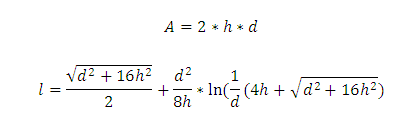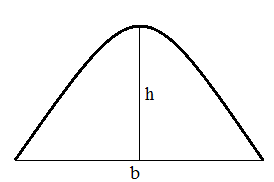# Segment of a Parabola Calculator

This CalcTown calculator calculates the area and arc-length of the segment of a parabola.

#### Result

m2
mClick here to view image

Where,

d = diameter of the segment

h = height of the segment

A = area of the segment

l = arc-length of the segment